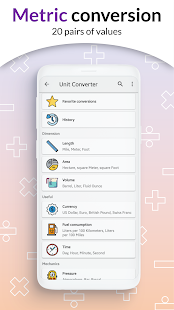This is all in one calculator, full mathematics function, working offline. A scientific calculator supports most of the features of fx 500, fx500, JX570ms, 570vnplus, 991es plus, 991ms, fx570esplus, fx-82 or TI-30XB, fx 4500, 82ms, 82es, fx-82AU PLUS II, 100AU, fx 4500pa, 991ex, fx 260.

This is all in one calculator, full mathematics function, working offline. A scientific calculator supports most of the features of fx 500, fx500, JX570ms, 570vnplus, 991es plus, 991ms, fx570esplus, fx-82 or TI-30XB, fx 4500, 82ms, 82es, fx-82AU PLUS II, 100AU, fx 4500pa, 991ex, fx 260.

★ Natural display ★
Natural Input allowing you to enter equations as you’d write them on paper, using fractions, roots, exponents and more. It’s called a “natural display” and is a common feature on scientific calculators like the fx 570 991 es plus!.

★ Scroll feature ★
Swipe on the keyboard to move the cursor up and down, left and right. Touch to edit expression.

★ Calculate functionality, advanced calculator feature ★
• Solve equation (Shift solve)
• CALC function (with memory function, 8 variable)
• Derivative function
• Integrate function
• Base N calculation (Calculator binary, decimal, hexadecimal, octal)
• Table generator: generate table base on expression.
• Solve system equation (two and three unknown variable)
• Calculate with fraction result (fraction calculator)
• Calculate with big numbers
• Complex number
• Matrix calculator with full feature of algebra calculator
• Percent % calculator
• logarithms calculator (log base n)

★ Angle unit ★

★ Output type ★
• Fraction (1/2)
• Mixed fraction (4/3 = 1 3/4)
• Decimal (1.23123)
• Repeat decimal (1.23232323 = 1.(23))
• Degrees/Minutes/Second
• Polar coordinate (Pol, Rec function)

★ Decimal Notation ★
Normal, Engineering, Engineering (SI), Science, Fixed

★ More feature ★
• Automatic calculate: see result as you typing (turn off in setting)
• Vibrate, vibrate strength
• Play sound when click sound button
• Decimal formatter
• Calculation history
• Show variable value
• Two layout: full keyboard and compact keyboard
• Change font family
• Change theme
• Change font size
• Copy, paste expression (long click to copy)

http://osewnui.oneskyapp.com/collaboration/translate/project/project/259731

★ Natural Scientific Calculator for Android ★

What’s New

# 3.4.7

• Add math formulas to favorite
• 1000+ Math formulas
• Fixed some bugs and minor improvements

Screenshots

•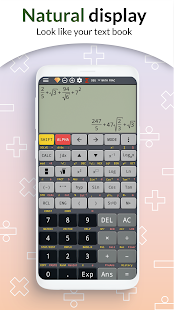•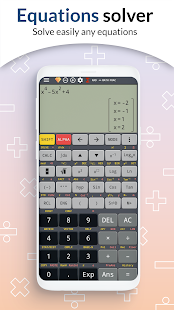•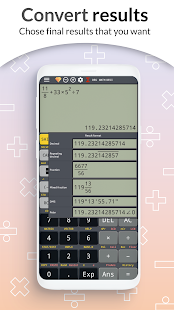•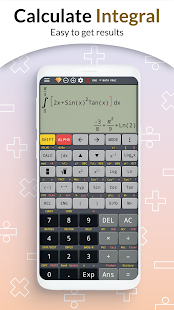•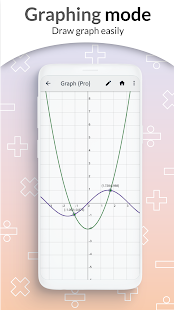•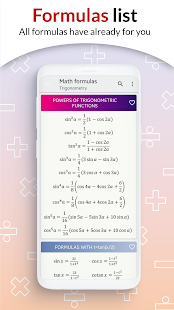•